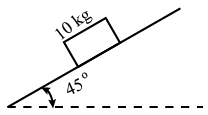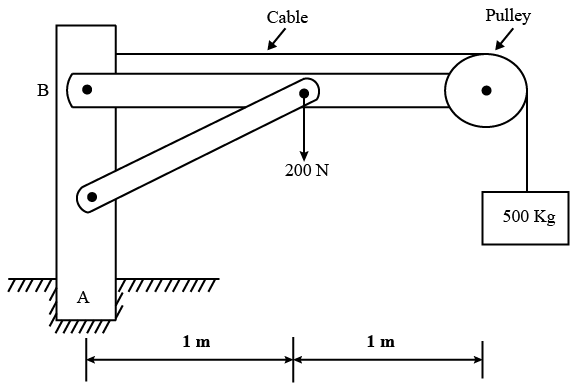# ISRO Scientist or Engineer Mechanical May 2017

Instructions

For the following questions answer them individually

Question 61

# Two Carnot heat engines are operating in series such that the heat sink of the first engine serves as the heat source of the second one. If the source temperature of the first engine is $$1327^\circ C$$, the sink temperature of the second engine is $$127^\circ C$$, and the thermal efficiency of both the engines are the same, the temperature of the intermediate reservoir is:Question 62

# A body of mass 10 kg is initially stationary on a $$45^\circ$$ inclined plane as shown in figure. The coefficient of dynamic friction between the body and the plane is 0.3. The body slides down the plane and attains a velocity of 20 m/s. The distance travelled (in metre) by the body along the plane is close toQuestion 63

# In metal forming, ring compression test is carried out to find outQuestion 64

# Water flows into the top of an open drum at a constant mass flow rate. Water exits through a pipe near the base with a mass flow rate proportional to the height of the liquid. If the drum is initially empty, which of the following gives the plot of liquid height with time?Question 65

# What is the approximate reaction moment at the fixed support A for the loaded frame shown below? (Take the diameter of the pulley as 250 mm)Question 66

# A radiator on a space capsule must dissipate heat generated inside the capsule, by radiating it into space. While on the bright side of earth, the capsule sees the earth’s radiation and the solar radiation. The radiator surface has a solar absorptivity of 0.5 and an emissivity of 0.9. What is the maximum equilibrium surface temperature of the radiator, if the maximum heat generated in the capsule is 800 W/m$$^2$$? Assume earth’s radiation = 100 W/m$$^2$$ and solar irradiation = 524 W/m$$^2$$.Take Stefan Boltzmann constant as $$5 \times 10^{-8} W/m^2-K^4$$Question 67

# For profile controls, datum isQuestion 68

# A simply supported beam of length L is subjected to a varying distributed load $$\sin \left(\frac{\pi x}{L}\right) N/m$$, where distance x is measured from the left support. The magnitude of the vertical reaction in N at the left support isQuestion 69

# A packed bed of solid spheres acts as a thermal energy storage system. In a charging process, heat transfer from the hot gas increases thermal energy stored within the spheres. Consider two beds, one with aluminium spheres ($$\rho = 2700 kg/m^3$$, C = 950 J/kg-K, k = 240 W/m-K) and other with copper spheres ($$\rho = 8900 kg/m^3$$, C = 400 J/kg-K, k = 380 W/m-K). Select the correct graph for the temperature rise of a sphere at the inlet of the bedQuestion 70

# Compressive residual stresses in weld jointsOR